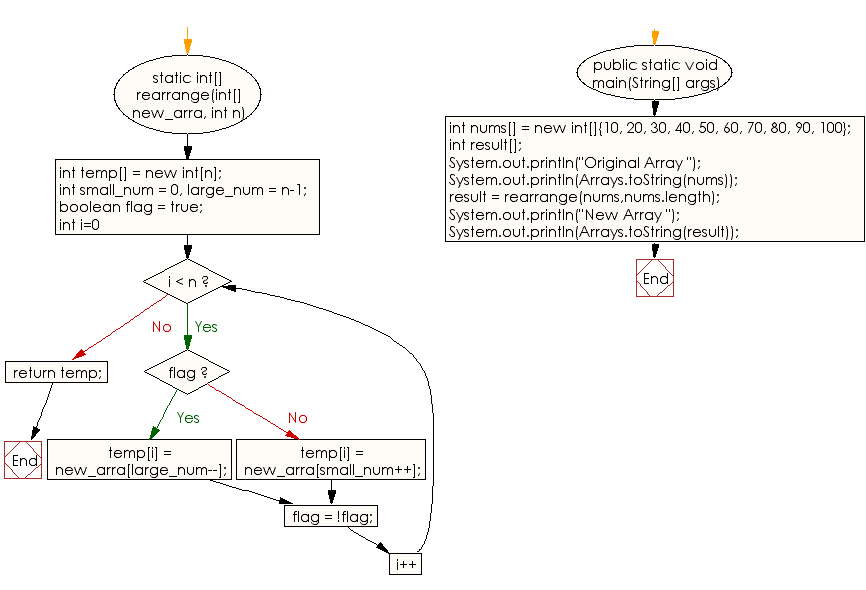﻿ Java: Positive integers of an array sorted in given pattern# Java Array Exercises: Sort an array of positive integers of a given array in the specified pattern

## Java Array: Exercise-50 with Solution

Write a Java program to sort an array of positive integers of a given array, in the sorted array the value of the first element should be maximum, second value should be minimum value, third should be second maximum, fourth second be second minimum and so on.

Sample Pattern:

```[100, 10, 90, 20, 80, 30, 70, 40, 60, 50]
```

Sample Solution:

Java Code:

``````import java.util.Arrays;

public class Main
{
static int[] rearrange(int[] new_arra, int n)
{
int temp[] = new int[n];

int small_num = 0, large_num = n-1;
boolean flag = true;

for (int i=0; i < n; i++)
{
if (flag)
temp[i] = new_arra[large_num--];
else
temp[i] = new_arra[small_num++];

flag = !flag;
}

return temp;
}

public static void main(String[] args)
{
int nums[] = new int[]{10, 20, 30, 40, 50, 60, 70, 80, 90, 100};
int result[];

System.out.println("Original Array ");
System.out.println(Arrays.toString(nums));

result = rearrange(nums,nums.length);

System.out.println("New Array ");
System.out.println(Arrays.toString(result));

}
}
```
```

Sample Output:

```
Original Array
[10, 20, 30, 40, 50, 60, 70, 80, 90, 100]
New Array
[100, 10, 90, 20, 80, 30, 70, 40, 60, 50]
```

Flowchart:Visualize Java code execution (Python Tutor):

Java Code Editor:

Improve this sample solution and post your code through Disqus

What is the difficulty level of this exercise?

Test your Programming skills with w3resource's quiz.

﻿

## Java: Tips of the Day

countOccurrences

Counts the occurrences of a value in an array.

Use Arrays.stream().filter().count() to count total number of values that equals the specified value.

```public static long countOccurrences(int[] numbers, int value) {
return Arrays.stream(numbers)
.filter(number -> number == value)
.count();
}
```

Ref: https://bit.ly/3kCAgLb